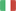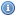EnglishItalianoVisitatore
Torna allaHomepage...

###Generali:

• Dipartimento: Ingegneria
• Tipologia: Corso Di Laurea Dm.270/04
• Corso di Laurea: Ingegneria Informatica
• Settore Ministeriale: MAT/05
• Codice di verbalizzazione: 8037591
• Metodi di insegnamento: Laboratorio
• Metodi di valutazione: Scritto
• Prerequisiti: Knowledge of the program of a standard "Analysis I" course.1) compute the limit of a real funcion on one variable 2) to analyze the grafh on a real function of one variable 3) compute the derivatives and integrals a real function of one vriable 4) be able to study the bahavior of a real function of one variable in the neighborhood of a point and in particular how to use the Taylor expansion both with Peano and Lagrange "Rests" 5) be able to compute the limit of real functions of more than one variable 6) c ompute the partial derivatives 7) be able to establish the presence of the tangent plane wich is equivalent to the difefrentiability of the function.
• Obiettivi: The students are supposed to be able to handle the matematical tecniques learnt during the course. In particular they are supposed to 1) integrate a real function of more than one variable 2) to be able recognize whether a vector field is conservative or not 3) be able to compute "line-integrals" that is integrals over a curve of the first and second type (difefrential forms) 4) be able to work with holomorohic functions and in general with the complex variables 5) be able to handle the Laplace transform and in particulr to solve the linear differential equations and systems of linear differential equations with constant coefficients
• Ricevimento: Su appuntamento da fissarsi tramite posta elettronica

###Didattica:

• A.A.: 2019/2020
• Canale: UNICO
• Crediti (CFU): 6
• Obbligo di Frequenza: No